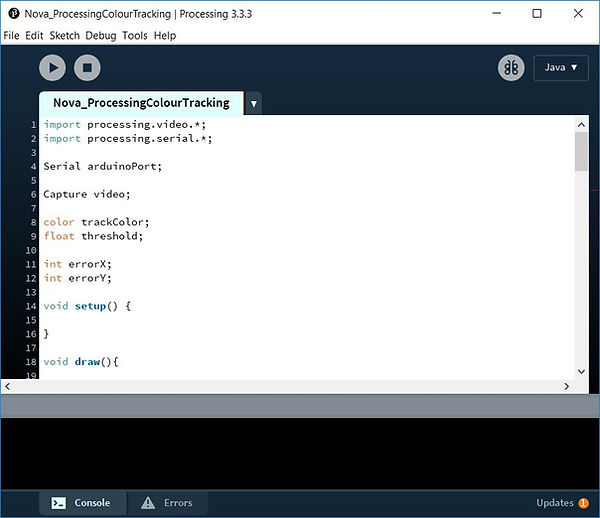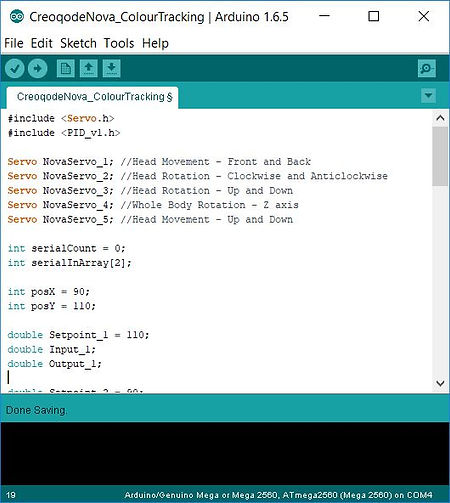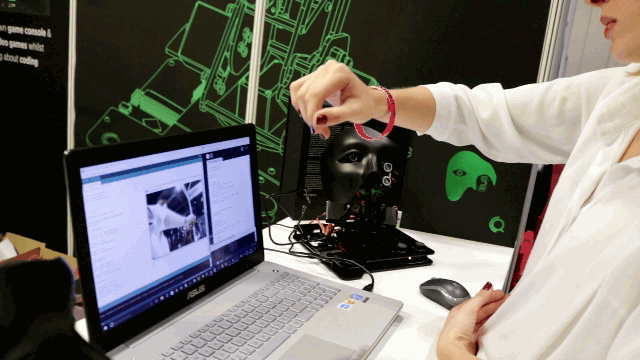## WITH NOVAColour tracking is another exciting computer vision project that you can do with Nova. There are many useful applications of colour tracking, as it allows you to track a single object with a specific colour amongst many other similar objects with the same shape and size. In such cases, identifying an object through its size and shape might be challenging, whereas identifying it through its colour is much more effective and requires less processing power.

The algorithm we are going to build for this tutorial is pretty simple. When we start capturing the video through Nova's camera, we will click on the colour we want Nova to track. Then according to the colour information (R, G, B values) of the pixel we clicked on, our PC will start scanning to find other pixels of the frame with similar colours. Depending on the frame rate you will be working with (this depends on the performance of your PC), PC will scan every single pixel in each frame of the video you are capturing. For this tutorial, we will be working with a frame rate of 15 frames per second and a resolution of 640 pixels by 360 pixels. This means your PC will scan 640 x 360 x 15 pixels, which is 3,456,000 pixels to scan in a second!

While this number sounds extreme, your PC will do this scanning without any problems. After scanning each frame, our sketch will return the average position of the pixels with the similar colour in (x, y) format. For this tutorial, x will vary between 0 and 640, and y between 0 and 360. Then, we will send this average position to Nova, and program it to move in such a way so that the average position of the colour we are tracking always remains at the centre of the frame.

Let's start by creating our sketch on Processing IDE. As described above, our main aim is to get the average position of a specific colour that we want to track. Start by adding the below lines before setup() function.

import processing.video.*;
import processing.serial.*;

Serial arduinoPort;

Capture video;

color trackColor;
float threshold;

int errorX;
int errorY;In the above lines, "errorX" and "errorY" are the variables where will be saving the difference between the average position of the tracked colour and the centre of the frame. These variables are also the ones that will be sent to Nova in order to tell it how to move so that the average position remains in the centre.

Threshold is the variable that you can change depending on the accuracy you want. As described above, we will be clicking on a pixel to identify the colour that will be tracked. However, it would be challenging if we were looking for the exact same R, G and B values. Even if it is the same object and the same colour, depending on the lighting of the ambience, there will be slightly different R, G and B values. As a solution, according to the threshold you will determine, your PC will consider similar colours as the one we want to track.

Let's move on to the setup() function.

size(640, 360);

String[] cameras = Capture.list();

printArray(cameras);

video = new Capture(this, cameras);
video.start();

trackColor = color(255, 0, 0);

arduinoPort = new Serial(this, "COM14", 9600);
arduinoPort.bufferUntil('\n');For this this tutorial, we will be using a resolution of 640 pixels by 360 pixels. Also, do not forget to change the COM port to the one your PC assigns to Nova.

We will now create a void function to be able to read from the video piece we will be capturing. Then, we will be starting to build the draw() function.

void captureEvent(Capture video) {
}

void draw() {

image(video, 0, 0);

threshold = 40; //You can change this value depending on the accuracy required

int avgX = 0;
int avgY = 0;

int count = 0;We are now going to build our "for loop" in order to scan each pixel in a frame.

This algorithm will use Euclidean distance theory, which is used to find the distance between two points in a three dimensional space. We are going to implement this method to find the distance between two colours by imagining their R, G and B values as their coordinates in a 3D space. If the distance between (R1, G1, B1) and (R2, G2, B2) is greater than the threshold defined, we will not consider that pixel as the one we are trying to track. However, if the distance between these colour values are less than the threshold, we will consider that colour as the one we are trying to track as well.

Copy the code lines below in your draw() function.

//Scan every pixel in the frame & find similar colours within threshold

for (int x = 0; x < video.width; x++ ) {
for (int y = 0; y < video.height; y++ ) {

int loc = x + y * video.width;
color currentColor = video.pixels[loc];

float r1 = red(currentColor);
float g1 = green(currentColor);
float b1 = blue(currentColor);

float r2 = red(trackColor);
float g2 = green(trackColor);
float b2 = blue(trackColor);

float d = distSq(r1, g1, b1, r2, g2, b2);

if (d < threshold*threshold) {
stroke(255);
strokeWeight(1);
point(x, y);
avgX += x;
avgY += y;
count++;
}
}
}Notice that the variable "count" is increased by one, if the colour distance of a scanned pixel is less than the threshold. Then, the position of that pixel is added to the previous average, and divided by the updated "count". This method will provide the exact average position of the colour we want to track in the frame.

if (count > 0) {

avgX = avgX / count;
avgY = avgY / count;

// Draw a circle at the average position of the tracked colour
fill(255);
strokeWeight(4.0);
stroke(0);
ellipse(avgX, avgY, 24, 24);

errorX = avgX/4;
errorY = avgY/2;

arduinoPort.write(errorX);
arduinoPort.write(errorY);
}
}

float distSq(float x1, float y1, float z1, float x2, float y2, float z2) {

float d = (x2-x1)*(x2-x1) + (y2-y1)*(y2-y1) + (z2-z1)*(z2-z1);
return d;

}Finally, we need to create the function which will allow us to pick the colour to be tracked with the click of a mouse.

void mousePressed() {

int loc = mouseX + mouseY*video.width;
trackColor = video.pixels[loc];

}That's it! We are done with the Processing IDE.

Now, connect the camera of Nova to your PC. Make sure it is the camera which is enabled by your PC and not the built-in camera. You can disable the built-in camera through Device Manager on Windows. This is explained in detail in the Face Tracking chapter. Also make sure you have changed the COM port at the beginning of this sketch, which must be the one you are using to upload sketches to Nova.

It is time to open Arduino IDE. We are going to create the sketch that tells Nova what to do according to the data it receives from the PC through Processing IDE. Add the lines below before your setup() function.

#include <Servo.h>
#include <PID_v1.h>

Servo NovaServo_1;      //Head Movement - Front and Back
Servo NovaServo_2;      //Head Rotation - Clockwise and Anticlockwise
Servo NovaServo_3;      //Head Rotation - Up and Down
Servo NovaServo_4;      //Whole Body Rotation - Z axis
Servo NovaServo_5;      //Head Movement - Up and Down

int serialCount = 0;
int serialInArray;

int posX = 90;
int posY = 110;

double Setpoint_1 = 110;
double Input_1;
double Output_1;

double Setpoint_2 = 90;
double Input_2;
double Output_2;

double Kp_1 = 0.016;
double Ki_1 = 0.012;
double Kd_1 = 0;

double Kp_2 = 0.028;
double Ki_2 = 0.026;
double Kd_2 = 0;

PID PID1(&Input_1, &Output_1, &Setpoint_1, Kp_1, Ki_1, Kd_1, DIRECT);
PID PID2(&Input_2, &Output_2, &Setpoint_2, Kp_2, Ki_2, Kd_2, DIRECT);As you will notice above, we are creating 2 PID controllers for the 2 servos we will be using for tracking colours. The values of Kp, Ki and Kd for both PID controllers work perfectly fine for us, but might need tuning for your Nova. During the assembly, any loose or strongly tighten parts will show different movement characteristics for Nova, thus the K coefficients will be different as well. Give it a try with the above values and see if you need further tuning.

Let's continue with the setup() function.

Serial.begin(9600);

NovaServo_3.attach(36);
NovaServo_4.attach(38);

NovaServo_3.write(110);
NovaServo_4.write(90);

PID1.SetMode(AUTOMATIC);
PID1.SetSampleTime(1);
PID1.SetOutputLimits(-35, 35);

PID2.SetMode(AUTOMATIC);
PID2.SetSampleTime(1);
PID2.SetOutputLimits(-35, 35);The function you see above, ".SetOutputLimits" is important for this case as it will prevent Nova from oscillating due to fast movements when the error is large. Regardless of the difference between "Setpoint" and "Input", the generated "Output" will not exceed -35 or 35.

Now let's move to the loop() function.

while(Serial.available() == 0);

serialCount++;

if (serialCount > 1){

Setpoint_1 = 110;
Input_1 = serialInArray;

Setpoint_2 = 90;
Input_2 = serialInArray;

PID1.Compute();
PID2.Compute();

posX = posX + Output_2;
posY = posY + Output_1;

NovaServo_4.write(posX);
if(posY > 75)NovaServo_3.write(posY);

serialCount = 0;
}First, we are programming Nova to look whether there is data coming from PC or not. If there is, it saves the data (average X, average Y) to the array "serialInArray" which has 2 elements. Average position of X, which is received from the PC, is used as input for the first PID controller. Average position of Y is used as input for the second PID controller. Then, the generated outputs are added to the initial position of the servo shafts to move Nova such that the average position always remains in the centre of the frame.

Upload this sketch to Nova, then open Processing IDE. When you click "Run", a window will open showing the video captured by Nova's camera. Now, find an object with a strong colour that easily stands out from the background. Show it to the camera and click on it with your mouse. You will see the colour that is tracked on the window, and Nova will start tracking it!

That's it! Nova can now track colours.